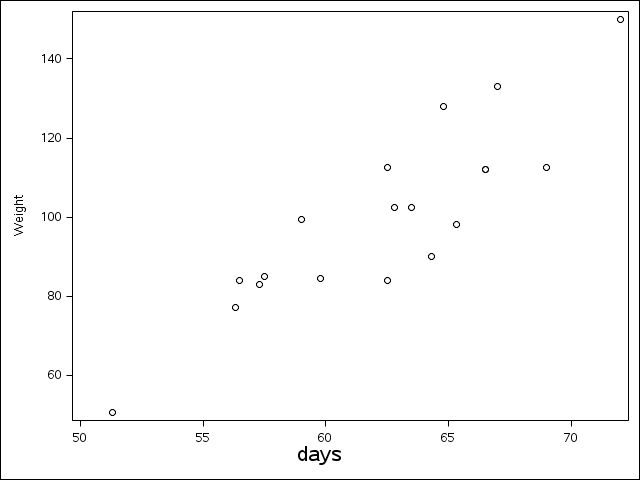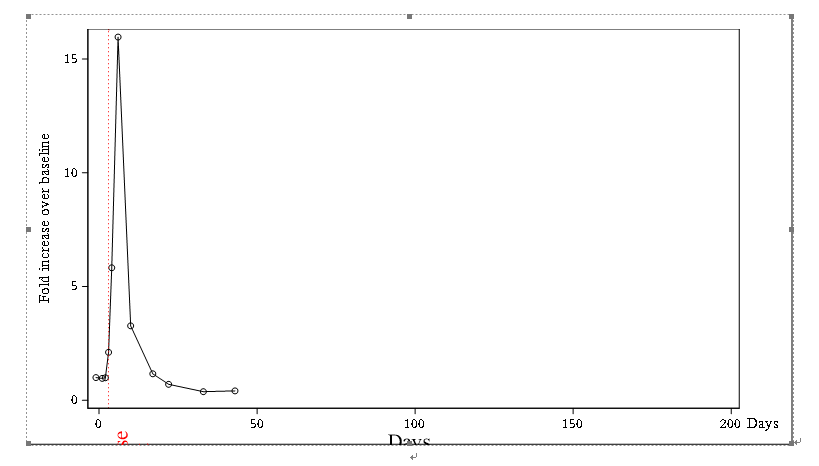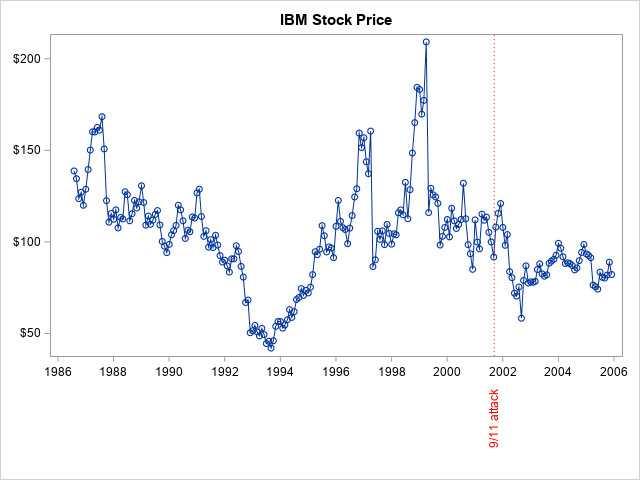## Reference line label and X-axis label are truncated in figure drawed by DRAWTEXT statement in GTL

I tried to add reference line label and X-axis label using drawtext statement in proc template in order to avoid overlapping with X-axis tick value. However the added labels are not displayed correctly which seems due to small space between figure border and x-axis.Could you help me figure out what's wrong with my code?

Here is my code,

/*--Define template--*/
proc template;

define statgraph RefLineLabel ;
begingraph;
entrytitle "";
layout overlay/XAXISOPTS=(linearopts=(viewmax=200) )
YAXISOPTS=(label='Fold increase over baseline');
seriesplot x=studyday y=aval2base / name='a' display=all;
referenceline x=eventday / /*curvelabel=event*/ lineattrs=(color=red pattern=dot)
curvelabellocation=outside CURVELABELPOSITION=auto curvelabelattrs=(color=red);
drawtext textattrs=(color=black size=15) "Days" /Y=0.5 x=50 yspace=graphpercent
xspace=graphpercent width=50 justify=center layer=front;
drawtext textattrs=(color=red size=15) "Adverse event" /Y=-5 x=3 xspace=datavalue yspace=datapercent
rotate=90 anchor=top width=15 justify=center;
endlayout;
endgraph;
end;
run;

/*--Create Graph--*/
proc sgrender data=example template=RefLineLabel;
run;

Here is my figure1 ACCEPTED SOLUTION

Accepted SolutionsDanH_sas
SAS Super FREQ

## Re: Reference line label and X-axis label are truncated in figure drawed by DRAWTEXT statement in GT

Whether you use DRAWTEXT with GTL or annotation with the SG procedures, the key is what @GraphGuy demonstrates in his example. Since you have the text value rotated you *MUST* also reserve space for the annotation, because the graph will not do that automatically, Think of annotation as an overlay (or underlay) of the actual graph. The PAD option @GraphGuy used with SGPLOT can also be used in GTL to reserve that space.

Hope this helps!

Dan

10 REPLIES 10

## Re: Reference line label and X-axis label are truncated in figure drawed by DRAWTEXT statement in GT

To label the X-axis you will likely be better off using the LABEL= option on the XAXISOPTS so the label text is included in the graph size determination. DRAWTEXT is applied over a graph and space is not allocated so you will likely spend a lot of time with each graph you make trying to force DRAWTEXT to create a nice enough label.

layout overlay/XAXISOPTS=(linearopts=(viewmax=200) label="Days" )
YAXISOPTS=(label='Fold increase over baseline');

You are most likely specifying the wrong coordinates for the DRAWTEXT for the reference line. You might investigate the CURVELABEL option for the referEnceline statement along with CURVELABELLOCATION=INSIDE and CURVELABELPOSITION

## Re: Reference line label and X-axis label are truncated in figure drawed by DRAWTEXT statement in GT

I'm looking at the example and I'm not sure why the x-axis label is being suppressed to begin with. The XAXISOPTS doesn't have a DISPLAY option listed, so I would have assumed it would print the x-axis label by default. To add to @ballardw if there is a reason to use drawtext for the label, but you still want the label space to be allocated you can make the x-axis label unbreakable spaces.

`````` proc template;
define statgraph blah;
begingraph;

layout overlay / xaxisopts=(label='A0A0A0'x);
scatterplot x=height y=weight;
drawtext textattrs=(color=black size=15) "days" / y=0 x=50 yspace=layoutpercent xspace=graphpercent width=50 justify=center
anchor=bottom layer=front;
endlayout;

endgraph;
end;
run;
ods graphics /reset;
proc sgrender data=sashelp.class template=blah;
run;``````## Re: Reference line label and X-axis label are truncated in figure drawed by DRAWTEXT statement in GT

@JeffMeyers wrote:

I'm looking at the example and I'm not sure why the x-axis label is being suppressed to begin with. The XAXISOPTS doesn't have a DISPLAY option listed, so I would have assumed it would print the x-axis label by default. To add to @ballardw if there is a reason to use drawtext for the label, but you still want the label space to be allocated you can make the x-axis label unbreakable spaces.

`````` proc template;
define statgraph blah;
begingraph;

layout overlay / xaxisopts=(label='A0A0A0'x);
scatterplot x=height y=weight;
drawtext textattrs=(color=black size=15) "days" / y=0 x=50 yspace=layoutpercent xspace=graphpercent width=50 justify=center
anchor=bottom layer=front;
endlayout;

endgraph;
end;
run;
ods graphics /reset;
proc sgrender data=sashelp.class template=blah;
run;``````What ASCII (or EBCDIC) character are you attempting to show with `'A0A0A0'x`?

xaxisopts=(label='45'x) will display an "E" as the label

``` proc template;
define statgraph blah;
begingraph;

layout overlay / xaxisopts=(labelattrs=(color=black size=15pt)label='Days' );
scatterplot x=height y=weight;
/*drawtext textattrs=(color=black size=15) "days" / y=0 x=50 yspace=layoutpercent xspace=graphpercent width=50 justify=center*/
/*   anchor=bottom layer=front;*/
endlayout;

endgraph;
end;
run;
ods graphics /reset;
proc sgrender data=sashelp.class template=blah;
run;```

## Re: Reference line label and X-axis label are truncated in figure drawed by DRAWTEXT statement in GT

Hello @ballardw, The 'A0'x is an unbreakable space. This is similar to a space in that it doesn't show anything (it's a blank space), but SAS considers it a character. In situations where a regular space would be ignored by SAS procedures an unbreakable space will be treated like any other character ('A', 'W', anything). In this case, when the label is set to be a blank (e.g. label=' ') I believe SAS ignores it and prints the x variable's label. But with an unbreakable space it leaves it alone so you can add a blank label and still allocate the graph space for it.

## Re: Reference line label and X-axis label are truncated in figure drawed by DRAWTEXT statement in GT

It still doesn't work when label options for reference line are off. I also tried changing yspace from datapercent to graphpercent in order to diaplay "Adverse event" properly below x-axis, however it doesn't work.

/*--Define template--*/
proc template;

define statgraph RefLineLabel ;
begingraph;
entrytitle "";
layout overlay/XAXISOPTS=(linearopts=(viewmax=200) )
YAXISOPTS=(label='Fold increase over baseline');
seriesplot x=studyday y=aval2base2 / name='a' display=all;
referenceline x=eventday / /*curvelabel=event*/ lineattrs=(color=red pattern=dot)
/*curvelabellocation=outside CURVELABELPOSITION=auto curvelabelattrs=(color=red)*/;
drawtext textattrs=(color=black size=15) "Days" /Y=0.5 x=50 yspace=graphpercent
xspace=graphpercent width=50 justify=center layer=front;
drawtext textattrs=(color=red size=15) "Adverse event" /Y=-5 x=3 xspace=datavalue yspace=graphpercent
rotate=90 anchor=top width=15 justify=center;
endlayout;
endgraph;
end;
run;

/*--Create Graph--*/
proc sgrender data=example template=RefLineLabel;
run;## Re: Reference line label and X-axis label are truncated in figure drawed by DRAWTEXT statement in GT

It sitll doesn't work when reference line label option is off.  I also tried changing yspace from datapercent to graphpercent and it didn't work.

/*--Define template--*/
proc template;

define statgraph RefLineLabel ;
begingraph;
entrytitle "";
layout overlay/XAXISOPTS=(linearopts=(viewmax=200) )
YAXISOPTS=(label='Fold increase over baseline');
seriesplot x=studyday y=aval2base2 / name='a' display=all;
referenceline x=eventday / /*curvelabel=event*/ lineattrs=(color=red pattern=dot)
/*curvelabellocation=outside CURVELABELPOSITION=auto curvelabelattrs=(color=red)*/;
drawtext textattrs=(color=black size=15) "Days" /Y=0.5 x=50 yspace=graphpercent
xspace=graphpercent width=50 justify=center layer=front;
drawtext textattrs=(color=red size=15) "Adverse event" /Y=-5 x=3 xspace=datavalue yspace=graphpercent
rotate=90 anchor=top width=15 justify=center;
endlayout;
endgraph;
end;
run;

/*--Create Graph--*/
proc sgrender data=example template=RefLineLabel;
run;

## Re: Reference line label and X-axis label are truncated in figure drawed by DRAWTEXT statement in GT

Perhaps rather than using GTL and proc sgrender, you could use proc sgplot and annotate the label?

Something like this ...

data anno_text;
length label \$100 anchor x1space y1space function \$50 textcolor \$12;
function='text';
x1space='datavalue'; y1space='datapercent';
textcolor='red'; textsize=9; textweight='normal';
width=100; widthunit='percent'; rotate=90;
x1='11sep2001'd; y1=-12; anchor='right'; label='9/11 attack'; output;
run;

title "IBM Stock Price";
proc sgplot data=sashelp.stocks (where=(stock='IBM')) pad=(bottom=20pct) sganno=anno_text;
series y=close x=date / markers;
yaxis display=(nolabel);
xaxis display=(nolabel);
refline '11sep2001'd / axis=x lineattrs=(color=red pattern=dot);
run;DanH_sas
SAS Super FREQ

## Re: Reference line label and X-axis label are truncated in figure drawed by DRAWTEXT statement in GT

Whether you use DRAWTEXT with GTL or annotation with the SG procedures, the key is what @GraphGuy demonstrates in his example. Since you have the text value rotated you *MUST* also reserve space for the annotation, because the graph will not do that automatically, Think of annotation as an overlay (or underlay) of the actual graph. The PAD option @GraphGuy used with SGPLOT can also be used in GTL to reserve that space.

Hope this helps!

Dan

## Re: Reference line label and X-axis label are truncated in figure drawed by DRAWTEXT statement in GT

Found the solution finally. It works when adding PAD option! Many thanks!

## Re: Reference line label and X-axis label are truncated in figure drawed by DRAWTEXT statement in GT

Thank you very much! It's a very good solution which inspire me how to reserve a space for annotation!

Discussion stats
• 10 replies
• 2765 views
• 2 likes
• 5 in conversation